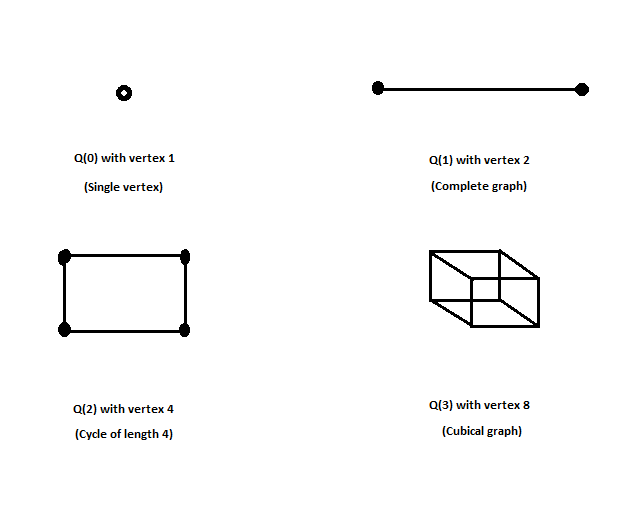# Hypercube Graph

You are given input as order of graph n (highest number of edges connected to a node), you have to find the number of vertices in a Hypercube graph of order n.

Examples:

```Input : n = 3
Output : 8

Input : n = 2
Output : 4
```

## Recommended: Please try your approach on {IDE} first, before moving on to the solution.

In hypercube graph Q(n), n represents the degree of the graph. Hypercube graph represents the maximum number of edges that can be connected to a graph to make it an n degree graph, every vertex has same degree n and in that representation, only a fixed number of edges and vertices are added as shown in the figure below:All hypercube graphs are Hamiltonian, hypercube graph of order n has (2^n) vertices, , for input n as the order of graph we have to find the corresponding power of 2.

## C++

 `// C++ program to find vertices in a hypercube  ` `// graph of order n ` `#include ` `using` `namespace` `std; ` ` `  `// function to find power of 2 ` `int` `power(``int` `n) ` `{ ` `    ``if` `(n == 1) ` `        ``return` `2; ` `    ``return` `2 * power(n - 1); ` `} ` ` `  `// driver program ` `int` `main() ` `{ ` `    ``// n is the order of the graph ` `    ``int` `n = 4; ` `    ``cout << power(n); ` `    ``return` `0; ` `} `

## Java

 `// Java program to find vertices in  ` `// a hypercube graph of order n  ` `class` `GfG ` `{ ` ` `  `    ``// Function to find power of 2  ` `    ``static` `int` `power(``int` `n)  ` `    ``{  ` `        ``if` `(n == ``1``)  ` `            ``return` `2``;  ` `        ``return` `2` `* power(n - ``1``);  ` `    ``}  ` `     `  `    ``// Driver program  ` `    ``public` `static` `void` `main(String []args) ` `    ``{ ` `         `  `        ``// n is the order of the graph  ` `        ``int` `n = ``4``; ` `        ``System.out.println(power(n)); ` `    ``} ` `} ` ` `  `// This code is contributed by Rituraj Jain `

## Python3

 `# Python3 program to find vertices in a hypercube  ` `#  graph of order n ` ` `  `# function to find power of 2 ` `def` `power(n): ` `    ``if` `n``=``=``1``: ` `        ``return` `2` `    ``return` `2``*``power(n``-``1``) ` ` `  ` `  `# Dricer code ` `n ``=``4` `print``(power(n)) ` ` `  ` `  `# This code is contributed by Shrikant13 `

## C#

 `// C# program to find vertices in  ` `// a hypercube graph of order n  ` `using` `System; ` ` `  `class` `GfG ` `{ ` ` `  `    ``// Function to find power of 2  ` `    ``static` `int` `power(``int` `n)  ` `    ``{  ` `        ``if` `(n == 1)  ` `            ``return` `2;  ` `        ``return` `2 * power(n - 1);  ` `    ``}  ` `     `  `    ``// Driver code  ` `    ``public` `static` `void` `Main() ` `    ``{ ` `         `  `        ``// n is the order of the graph  ` `        ``int` `n = 4; ` `        ``Console.WriteLine(power(n)); ` `    ``} ` `} ` ` `  `// This code is contributed by Mukul Singh `

## PHP

 ` `

Output:

```16
```

Don’t stop now and take your learning to the next level. Learn all the important concepts of Data Structures and Algorithms with the help of the most trusted course: DSA Self Paced. Become industry ready at a student-friendly price.

My Personal Notes arrow_drop_upCheck out this Author's contributed articles.

If you like GeeksforGeeks and would like to contribute, you can also write an article using contribute.geeksforgeeks.org or mail your article to contribute@geeksforgeeks.org. See your article appearing on the GeeksforGeeks main page and help other Geeks.

Please Improve this article if you find anything incorrect by clicking on the "Improve Article" button below.

Article Tags :
Practice Tags :

3

Please write to us at contribute@geeksforgeeks.org to report any issue with the above content.